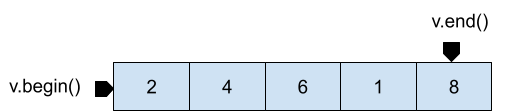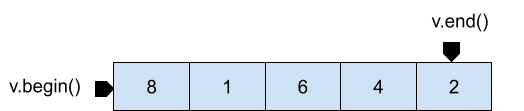×

Search anything:

# Different ways to reverse vector in C++ STL

#### C++ Software EngineeringIn this article, we take a look at different ways to reverse vector in C++ Standard Template Library (STL) along with reversing a 2D vector.

1. Introduction to Vector
2. C++ vector STL function std::reverse()
3. By swapping the elements
4. By using reverse iterators
5. Consider the case of a 2D vector
6. Implementation - reversing 2D vector rows
7. Implementation - reversing 2D vector columns

Pre-requisites:

## Introduction to Vector

A vector is a dynamically sized array that can resize itself automatically. Elements in a vector are stored in contiguous locations in memory. Vectors use random access iterators to access or navigate through the elements stored in a vector.

Before we dive into the different methods, let's recall the iterators vector::begin() and vector::end().begin() returns an iterator that points to the first element in the vector whereas end() returns an iterator that points just beyond the last element in the vector.

On reversal, the vector would look as follows :Note that reversing the vector does not mean an interchange in the location where the iterators begin() and end() point to, only the values stored in the locations to which the iterators point to are swapped.

There are 3 simple ways in which you can reverse a vector:

## 1. C++ vector STL function std::reverse()

std::reverse is a built-in function defined in the header file `<algorithm>` as

`template <class BidirectionalIterator>`
`void reverse (BidirectionalIterator first, BidirectionalIterator last)`

std::reverse() reverses the order of elements in the range [first,last).
The function takes two parameters - iterator pointing to the first element in the range and iterator pointing to the last element in the range. This function applies `std::iter_swap` for swapping the elements from both ends of the given range.

``````#include <algorithm>
#include <iostream>
#include <vector>
using namespace std;

int main()
{
vector<int> v={2,4,1,6,8};

cout<<"Original Vector: \n";
for(auto iter = v.begin(); iter < v.end(); iter++)
{
cout << *iter << " ";
}
cout <<"\n";

//std::reverse() function of STL
reverse(v.begin(),v.end());

cout<<"Reversed Vector:\n";
for (auto iter = v.begin(); iter < v.end(); iter++)
{
cout << *iter<< " ";
}
return 0;
}

``````

### Time and space Complexity

Since the number of swaps made internally is equal to (last-first)/2 [where first and last define the range of elements to reverse], the time complexity is given by O((last-first)/2))

Therefore, reversing an entire vector has a time complexity of O(n).

Space complexity is O(1) as no extra space is used to perform the swaps.

## 2. By swapping the elements

We can reverse a vector by traversing and swapping elements in the range v.begin() and v.end().

``````#include <iostream>
#include <vector>
#include <algorithm>
using namespace std;

int main()
{
vector<int> v = { 1, 2, 3, 4, 5 };
cout<<"Original Vector: \n";
for(auto iter = v.begin(); iter < v.end(); iter++)
{
cout << *iter << " ";
}
cout <<"\n";

// swapping
for (auto first = v.begin(),last=v.end()-1; first<last; first++,last--) {
swap(*first, *last);
}

cout<<"Reversed Vector:\n";
for (auto iter = v.begin(); iter < v.end(); iter++)
{
cout << *iter<< " ";
}
return 0;
}
``````

### Time and Space Complexity

Time complexity is O((last-first)/2) = O(n).
Space complexity is O(1) as no extra space is used.

## 3. By using reverse iterators

C++ vectors support reverse iterators vector::rbegin() and vector::rend().
rbegin() returns a reverse iterator pointing to the last element in the vector whereas rend() returns a reverse iterator pointing just in front of the first first element.

We can obtain the reverse of a vector by using range constructor to create a new vector with reverse iterators as parameters.

We can then swap the original vector with the new reversed vector by using vector::swap as `v.swap(v2)` or std::swap as `swap(v,v2)` or simply do `v=v2`

``````#include <algorithm>
#include <iostream>
#include <vector>
using namespace std;

int main()
{
vector<int> v={2,4,1,6,8};

cout<<"Original Vector: \n";
for(auto iter = v.begin(); iter < v.end(); iter++) {
cout << *iter << " ";
}
cout <<"\n";

vector<int> v2 (v.rbegin(),v.rend());

v.swap(v2);
cout<<"Reversed Vector:\n";
for(auto iter = v.begin(); iter < v.end(); iter++) {
cout << *iter << " ";
}

return 0;
}
``````

### Time and Space Complexity

Swapping two vectors has a constant time complexity O(1) as it only swaps the addresses of the two vectors.
The space complexity is O(n) as extra space is used to create new vector.

## Consider the case of a 2D vector

A 2D vector is a vector with every element as a vector with variable sizes.

Let's take the case of a 2D vector having 3 rows and 4 columns in each row, as shown below.On reversing the rows, we obtain the vector as shown below.Whereas on reversing the columns, we obtain the vector as shown below.## 1. Implementation - reversing 2D vector rows

Using `std::reverse()`

v.begin() returns an iterator pointing to the first row of the 2D vector and v.end() returns an iterator pointing to the last row.

A single line of code, `reverse(v.begin(),v.end())` is sufficient to reverse the vector. The function swaps the rows internally in the range [v.begin(),v.end()).

``````#include <algorithm>
#include <iostream>
#include <vector>

using namespace std;

int main()
{
vector<vector <int>> v {{1,2,3,4},{5,6,7,8},{9,10,11,12}};

for (int i = 0; i < v.size(); i++)
{
for (int j = 0; j < v[i].size(); j++)
{
cout << v[i][j] << " ";
}
cout <<"\n";
}

// swaps all the rows
reverse(v.begin(),v.end());

cout<<"After row reversal: \n";

for (int i = 0; i < v.size(); i++)
{
for (int j = 0; j < v[i].size(); j++)
{
cout << v[i][j] << " ";
}
cout <<"\n";
}

return 0;
}
``````

## 2. Implementation - reversing 2D vector columns

Using `std::reverse()`
To reverse the columns of a 2D vector, we need to traverse through the vector row-wise and reverse elements of each row.

v.size() returns the number of rows in the 2D vector.
Using a for loop, we traverse through each row and apply reverse(v[i].begin(), v[i].end()) to the ith row.

v[i].begin() returns an iterator that points to the first element in the ith row whereas, v[i].end() returns an iterator that points just beyond the last element in the ith row.

The function `reverse(v[i].begin(), v[i].end())` swaps elements from both ends of the ith row.

Following this, we print each element in the ith row by traversing through the row using a for loop.
Note that v[i].size() returns the number of columns in the ith row.

``````#include <algorithm>
#include <iostream>
#include <vector>

using namespace std;

int main()
{
vector<vector <int>> v {{1,2,3,4},{5,6,7,8},{9,10,11,12}};

for (int i = 0; i < v.size(); i++)
{
for (int j = 0; j < v[i].size(); j++)
{
cout << v[i][j] << " ";
}
cout <<"\n";
}
cout<<"After column reversal: \n";

for (int i = 0; i < v.size(); i++)
{
//reversing elements of each row
reverse(v[i].begin(),v[i].end());

for (int j = 0; j < v[i].size(); j++)
{
//displaying
cout << v[i][j] << " ";
}
cout <<"\n";
}

return 0;
}
``````

### Time Complexity

The time complexity of reversing columns as well as rows is O(n).

With this article at OpenGenus, you must have the complete idea of how to reverse a vector in C++ STL.